2019-03-27 21:06:50 kilotwo 阅读数 349

rows:行
cols:列

## 常用的数据结构

``````#include<opencv2\opencv.hpp>
#include<opencv2\highgui\highgui.hpp>

using namespace std;
using namespace cv;

//常见数据结构使用方法总结
int main()
{
//Mat的用法
Mat m1(2, 2, CV_8UC3, Scalar(0, 0, 255)); //其中的宏的解释：CV_[位数][带符号与否][类型前缀]C[通道数]
cout << m1 << endl;

//或者,利用IplImage指针来初始化,将IplImage*转化为Mat
Mat mat = cvarrToMat(image);

//Mat转IplImage:
IplImage img = IplImage(mat);

//或者
Mat m2;
m2.create(4, 5, CV_8UC(2));

//点的表示:Point
Point p;
p.x = 1; //x坐标
p.y = 1; //y坐标

//或者
Point p2(1, 1);

//颜色的表示：Scalar(b,g,r);注意不是rgb，注意对应关系
Scalar(1, 1, 1);

//尺寸的表示:Size
Size(5, 5);// 宽度和高度都是5

//矩形的表示：Rect，成员变量有x,y,width,height
Rect r1(0, 0, 100, 60);
Rect r2(10, 10, 100, 60);
Rect r3 = r1 | r2; //两个矩形求交集
Rect r4 = r1 & r2; //两个矩形求并集

waitKey(0);
``````

## 访问图片中像素的方式

Mat类有若干成员函数可以获取图像的属性。cols表示列，rows表示行，channels()返回图像的通道数，灰度图通道数1，彩色图通道数3。每行的像素值由一下语句得到：

``````int colNumber = outputImage.cols*outputImage*channels();//列数*通道数=每一行元素个数 （有点三维立体的感觉）
``````

``````  uchar* data = img.ptr<uchar>(i);  //获取第i行地址
``````
``````#include<opencv2\opencv.hpp>
#include<opencv2\highgui\highgui.hpp>

using namespace std;
using namespace cv;

//使用指针的方式
int main()
{
for (int i = 0; i < img.rows; i++)
{
uchar* data = img.ptr<uchar>(i);  //获取第i行地址
for (int j = 0; j < img.cols; j++)
{
printf("%d\n",data[j]);
}
}

waitKey(0);

}
``````

## 感兴趣区域：ROI

1.使用表示矩形区域的Rect
2.Range指定感兴趣的行或列的范围

## 基础图像操作

void namedWindow(const String& winname, int flags = WINDOW_AUTOSIZE);

namedWindow还有一个很重要的功能，如果使用默认参数，窗口是无法自由调整的，如果想实现用户自由拉伸窗口，可以这么做：

`````` namedWindow("srcImage", WINDOW_NORMAL);// 注意这个宏，使用WINDOW_NORMAL可以允许用户自由伸缩窗口大小
imshow("srcImage", srcImage);
``````

``````bool imwrite( const String& filename, InputArray img,const std::vector&params = std::vector());
``````

``````#include<opencv2\opencv.hpp>
#include<opencv2\highgui\highgui.hpp>

using namespace std;
using namespace cv;

#define PIC_MAX_NUM 5

int pic_num = 0;

void on_track(int,void*)
{
char file;
sprintf(file, "%d.jpg", pic_num);
if (!img.data)
{
cout << "读取图片失败" << endl;
return;
}
imshow("展示多幅图片", img);
}

int main()
{
namedWindow("展示多幅图片");
createTrackbar("图片编号", "展示多幅图片", &pic_num, PIC_MAX_NUM, on_track);
on_track(pic_num, NULL);
waitKey(0);

}
``````

## 转为灰度图

``````#include<opencv2\opencv.hpp>
#include<opencv2\highgui\highgui.hpp>

using namespace std;
using namespace cv;

int main()
{
Mat dstImg;
cvtColor(img, dstImg,COLOR_BGR2GRAY);//从宏名字就可以知道，是彩色图转换到灰度图
imshow("灰度图", dstImg);

waitKey(0);

}
``````

## 图像二值化操作

1.全局固定阈值很容易理解，就是对整幅图像都是用一个统一的阈值来进行二值化；

2.局部自适应阈值则是根据像素的邻域块的像素值分布来确定该像素位置上的二值化阈值。

``````#include<opencv2\opencv.hpp>
#include<opencv2\highgui\highgui.hpp>

using namespace std;
using namespace cv;

int main(int argc, char** argv)
{
if (image.empty())
{
cout << "read image failure" << endl;
return -1;
}

// 全局二值化
int th = 100;
Mat global;
threshold(image, global, th, 255, CV_THRESH_BINARY_INV);

// 局部二值化
int blockSize = 25;
int constValue = 10;
Mat local;

imshow("全局二值化", global);
imshow("局部二值化", local);

waitKey(0);
return 0;
}
``````

## Canny边缘检测

``````#include <iostream>
#include <opencv2\opencv.hpp>

using namespace cv;
using namespace std;
int main()
{
imshow("Src Pic", SrcPic);
Mat DstPic, edge, grayImage;

//创建与src同类型和同大小的矩阵
DstPic.create(SrcPic.size(), SrcPic.type());

//将原始图转化为灰度图
cvtColor(SrcPic, grayImage, COLOR_BGR2GRAY);

//先使用3*3内核来降噪 edge为均值滤波后图像，需与原图像大小一样
blur(grayImage, edge, Size(3, 3));

//运行canny算子，最后一个3 是默认算子孔径
Canny(edge, edge, 3, 9, 3);

imshow("边缘提取效果", edge);

waitKey();
return 0;
}

``````

## 直方图均衡化

``````#include<opencv2\opencv.hpp>
#include<opencv2\highgui\highgui.hpp>

using namespace std;
using namespace cv;

//直方图均衡化
int main()
{
imshow("原始图", img);
Mat dst;
cvtColor(img, img, CV_RGB2GRAY);
imshow("灰度图", img);
equalizeHist(img, dst);

imshow("直方图均衡化", dst);

waitKey(0);

}
``````

2018-12-24 13:50:24 linghugoolge 阅读数 1746

# 一、效果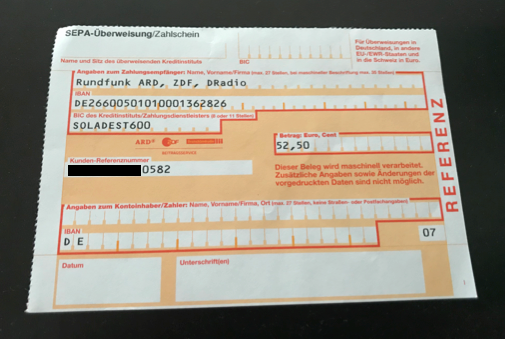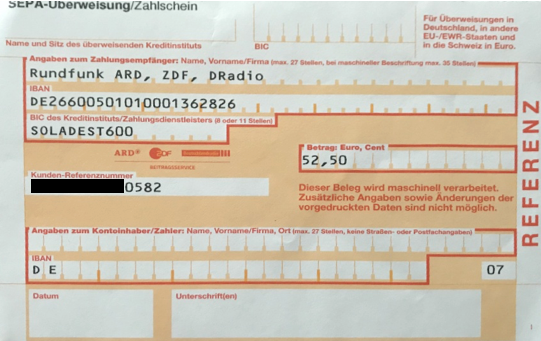# 二、代码实现

## 1、python代码，基于opencv库和imutils库

``````from imutils import perspective
from skimage.filters import threshold_local
import cv2
import imutils

# 边缘扫描
ratio = image.shape / 500.0                                 # 比例
orig = image.copy()
image = imutils.resize(image, height = 500)

# 灰度转换及边缘查找
gray = cv2.cvtColor(image, cv2.COLOR_BGR2GRAY)
gray = cv2.GaussianBlur(gray, (5, 5), 0)
edged = cv2.Canny(gray, 75, 200)                               # 边缘检测

# 只保留轮廓
cnts = cv2.findContours(edged.copy(), cv2.RETR_LIST, cv2.CHAIN_APPROX_SIMPLE)#通过边缘图像找到轮廓
cnts = cnts if imutils.is_cv2() else cnts                # 用以区分OpenCV2.4和OpenCV3
cnts = sorted(cnts, key = cv2.contourArea, reverse = True)[:5] # 保留最大轮廓

for c in cnts:
peri = cv2.arcLength(c, True)
approx = cv2.approxPolyDP(c, 0.02 * peri, True)            # 轮廓点
if len(approx) == 4:                                       # 表明找到四个轮廓点
screenCnt = approx
break

# 转为鸟瞰图
warped = perspective.four_point_transform(orig, screenCnt.reshape(4, 2) * ratio)
warped = cv2.cvtColor(warped, cv2.COLOR_BGR2GRAY)              # 灰度转换
T = threshold_local(warped, 11, offset = 10, method = "gaussian")
warped = (warped > T).astype("uint8") * 255

cv2.imshow("Original", imutils.resize(orig, height = 650))
cv2.imshow("Scanned", imutils.resize(warped, height = 650))
cv2.waitKey(0)
``````

## 2、C++实现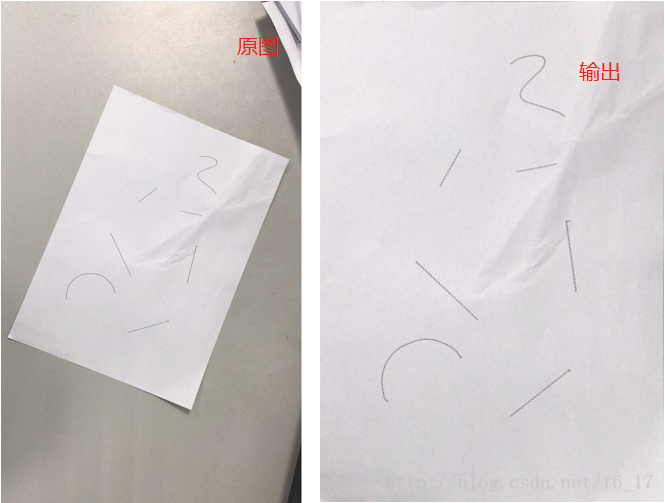## 3、Image perspective transformation and text recognition

•  Image cropping automation
•  Bird's eye view transformation
• Optical character recognition

2018-12-24 14:27:19 linghugoolge 阅读数 1752

# 一、效果

4个不同方向的相机，将其鸟瞰变化后，进行拼接，得到车辆及周围区域的鸟瞰视角图。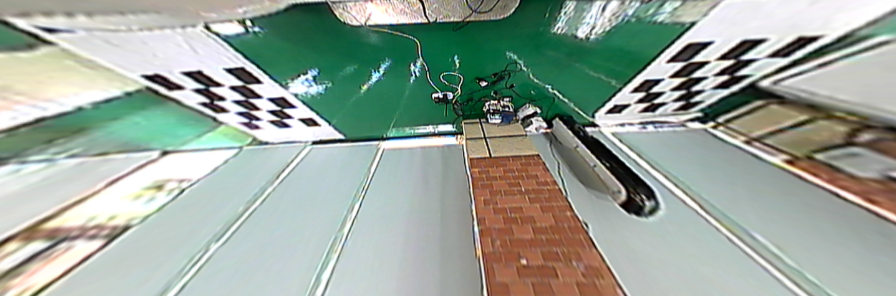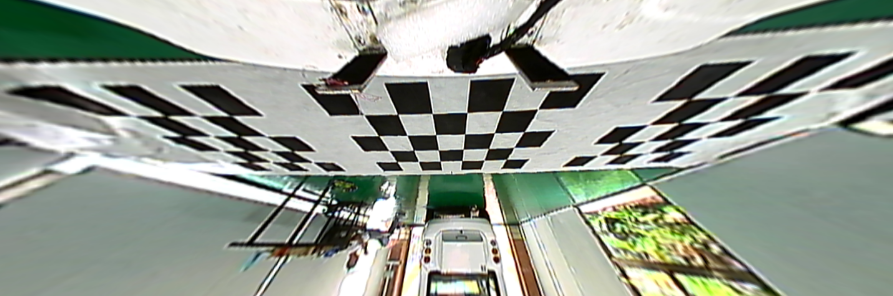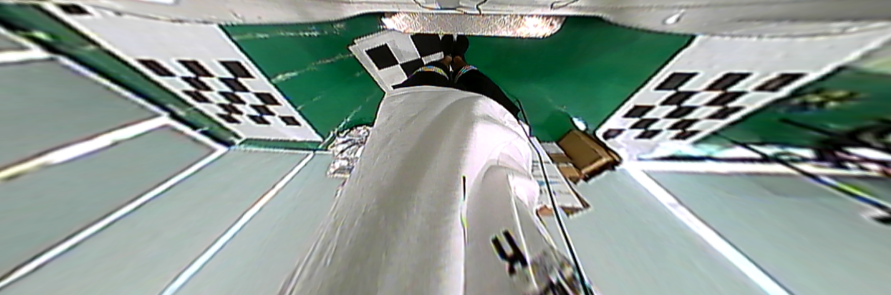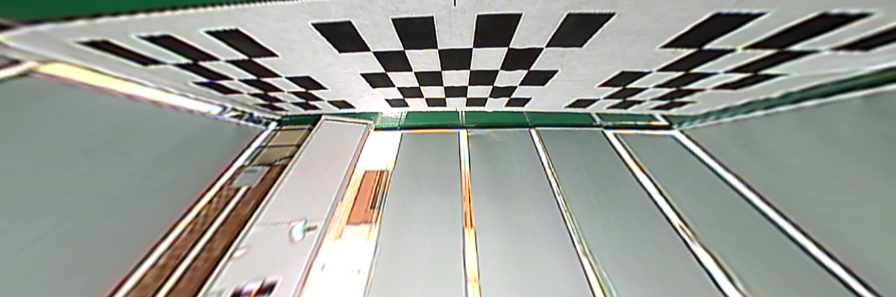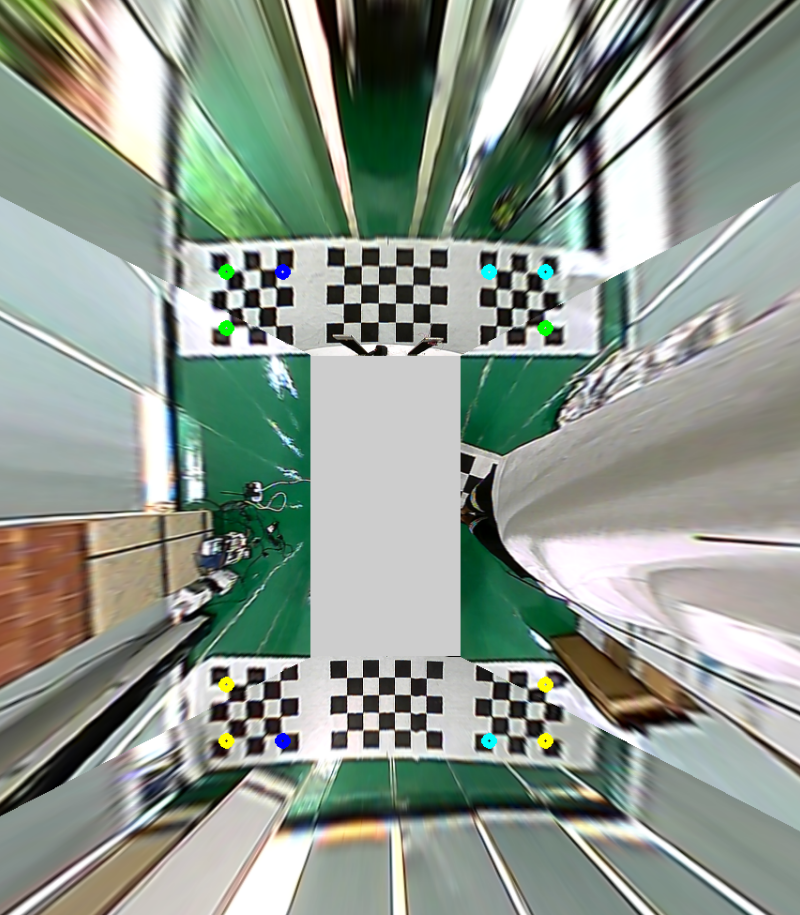1、相机的标定和图片校正；

2、图像拼接；

3、拼接缝消除；

4、移植到FPGA。

# 三、代码

``````#include  "birdView.hpp"

int main(){
Mat v;

for (int i = 0; i < 4; i++){
char buf;
sprintf(buf, "%d.png", i);
}

BirdView b("config.yml");

b.setCarSize(240, 380);
b.setChessSize(60);
b.setInternalShift(27,27);

//b.sourcePointClick(v);

while (1){
imshow("bird view", b.transformView(v));
if (waitKey(20) == 27)	break;
}
}``````

2018-03-03 15:23:21 w77AYU 阅读数 4480

透视变换常用于图像视觉处理中，如在移动机器人视觉系统中，摄像机光轴与地面并不是呈垂直关系，而是有一定的倾斜角度，因此想要获取俯视图即正投影的效果，就需要对图像进行透视变换。进行透视变换获取俯视图的常用方法有两种：1、基于图像的单应性矩阵DLT算法。2、基于摄像机倾斜角度的变换，也就是上文提到的把世界坐标系转为摄像机坐标系的过程。由于在实际中我们比较难以准确测到摄像机的倾斜角度，因此我们采用第一种方法来进行透视变换获取俯视图。两个不同视角的图像上的点对的可以用一个射影变换表述，即：，有：图像配准图像融合是图像拼接的两个关键技术。图像配准是图像融合的基础,而且图像配准算法的计算量一般非常大,因此图像拼接技术的发展很大程度上取决于图像配准技术的创新 ,早期的图像配准技术主要采用点匹配法,这类方法速度慢、精度低,而且常常需要人工选取初始匹配点,无法适应大数据量图像的融合。图像拼接的方法很多,不同的算法步骤会有一定差异,但大致的过程是相同的。一般来说,图像拼接主要包括以下四步:图像预处理、建立变换模型、统一坐标变换、融合重构。

图像配准根据不同配准原理，主要可以分为三种配准方法：基于图像亮度信息的配准方法、基于特征的图像配准方法、基于变换域的图像配准方法。

以上三种配准方法都是根据图像之间重合区域信息来自动配准的，一般自动配准的方法运算量大，精确度不高。本车载环视拼接系统对实时性要求高，自动配准方法不利于系统实现。而且对于车载环视系统来说，每个摄像头都固定在车上，他们之间的相对位置不会改变，因此我们采用手动选取参考点实现配准的方法。

在全景俯视图中，相邻两方向俯视图之间存在一定的重合区域，另外由于我们求解单应性矩阵时对四个区域方向选取了参考点，因此我们可以在选取参考点时选取相邻两幅畸变校正图的两个重合点作为求解单应性矩阵的参考点，即相邻图像选取共同参考点，并且在全景俯视图下建立统一的坐标系，如图所示。这样当四路视频图像经透视变换后映射到各自相应俯视图区域即完成图像配准，这样就可以把四个方向图像在侧视图变成俯视图时拼接起来。(1) 相邻两视频区域拼接处有明显的拼接缝。

(2) 四路视频在亮度上有较大的差异，存在亮暗不均一的情况。

(3)从某一视频区域过渡到其相邻视频区域拼接处有折断现象。

2018-12-04 16:03:55 lyq_12 阅读数 145

①、图像分辨率：864×480；

②、行滤波模板系数选取：

float arryFilter[]={-0.125, -0.125, -0.125, -0.125, 0.25, 0.25, 0.25, 0.25, -0.125, -0.125, -0.125, -0.125};

③、行滤波结果处理：

if (sum < 0.1)

{sum = 0;}

int int_sum = (int)sum;

outGrayImg.at<uchar>(i,j) = (uchar)int_sum;

④、行滤波效果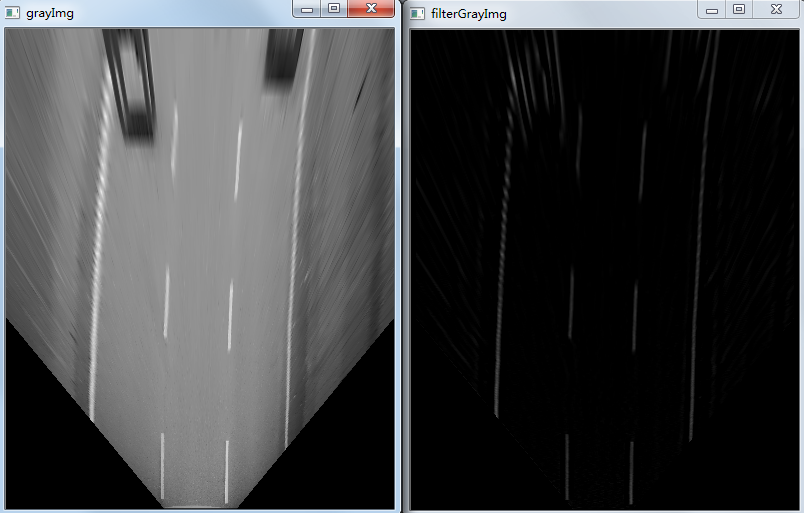图(a)                                                                         图(b)